NEET  >  NEET Previous Year Questions (2014-22): Mechanical Properties of Solids

# NEET Previous Year Questions (2014-22): Mechanical Properties of Solids - Notes | Study Physics Class 11 - NEET

 1 Crore+ students have signed up on EduRev. Have you?

Q.1. Given below are two statements: One is labelled as Assertion (A) and the other is labelled as Reason (R).
Assertion (A): The stretching of a spring is determined by the shear modulus of the material of the spring.
Reason (R): A coil spring of copper has more tensile strength than a steel spring of same dimensions. In the light of the above statements, choose the most appropriate answer from the options given below
(1) Both (A) and (R) are true and (R) is not the correct explanation of (A)
(2) (A) is true but (R) is false
(3) (A) is false but (R) is true
(4) Both (A) and (R) are true and (R) is the correct explanation of (A)                   
Sol. It is true that the stretching of spring is determined by shear modulus of the spring as when coil spring is stretched neither its length nor its volume changes, there is only change in its shape. The tensile strength of steel is more than that of copper. Hence Assertion is true and reason is false.

Q.2. Dimensions of stress are :     
A: [ML0T–2]
B: [ML–1T–2]
C: [MLT2]
D: [ML2T–2]
Ans:
B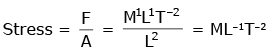Q.3. A wire of length L, area of cross-section A is hanging from a fixed support. The length of the wire changes to L1 when mass M is suspended from its free end. The expression for Young's modulus is :     
A: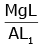B: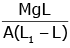C: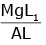D: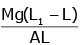Ans: B
from young’s modulus formula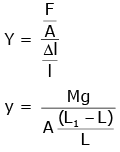Q.4. When a block of mass M is suspended by a long wire of length L, the length of the wire becomes (L + l). The elastic potential energy stored in the extended wire is:    
A: Mgl
B: MgL
C: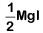D: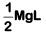Ans:
C
Solution: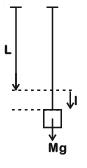U = 1/2 ((work done by gravity)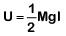Q.5. Two wires are made of the same material and have the same volume. The first wire has cross-sectional area A and the second wire has cross-sectional area 3A. If the length of the first wire is increased by Δl on applying a force F, how much force is needed to stretch the second wire by the same amount ?    
A: 9F
B: 6F
C: 4F
D: F
Ans:
A
Solution: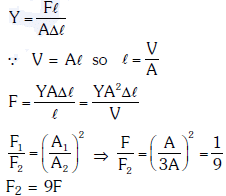Q.6. The bulk modulus of a spherical object is 'B'. If it is subjected to uniform pressure 'p', the fractional decrease in radius is:-    
A: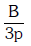B: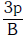C: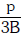D: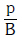Ans:
C
Solution: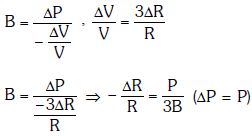Q.7. Copper of fixed volume ‘V’ is drawn into wire of length ‘l’. When this wire is subjected to a constant force ‘F’, the extension produced in the wire is ‘Δl’. Which of the following graphs is straight line?    
A: Δl versus l/l2
B: Δl versus l
C: Δl versus l/l
D: Δl versus l2
Ans:
D
Solution: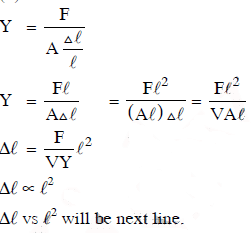There is no Question for NEET 2021

The document NEET Previous Year Questions (2014-22): Mechanical Properties of Solids - Notes | Study Physics Class 11 - NEET is a part of the NEET Course Physics Class 11.
All you need of NEET at this link: NEET

## Physics Class 11

127 videos|464 docs|210 tests
 Use Code STAYHOME200 and get INR 200 additional OFF

## Physics Class 11

127 videos|464 docs|210 tests

### How to Prepare for NEET

Read our guide to prepare for NEET which is created by Toppers & the best Teachers

Track your progress, build streaks, highlight & save important lessons and more!

,

,

,

,

,

,

,

,

,

,

,

,

,

,

,

,

,

,

,

,

,

;